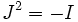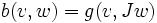# Almost Hermitian structure gives symplectic form

## Statement

Let$M$ be a differential manifold,$J$ be an almost complex structure on$M$ (viz a smooth choice of map from each tangent space to itself such that$J^2 = -I$). Let$g$ be a Riemannian metric on$M$, such that:$g(v,Jw) + g(Jv,w) = 0$

The tripe$(M,g,J)$ is an almost Hermitian structure on$M$. This gives rise to the following almost symplectic structure: the bilinear form on a tangent space is defined as:$b(v,w) = g(v,Jw)$

## Interpretation in terms of structure groups

An almost Hermitian structure is a reduction ofthe structure group of the$2n$-dimensional manifold to$U(n,\mathbb{C})$, while an almost symplectic structure is a reduction of the stucture group to$Sp(2n,\mathbb{R})$. Since$U(n,\mathbb{C})$ is a subgroup of$Sp(2n,\R)$ an almost Hermitian structure gives an almost symplectic structure.